### Calculate tract based weighted means

Extracting the weighted means of individual fiber pathways can be useful when you want to quantify the microstructure of an entire white matter structure. This is specifically useful for tract based analyses, where you run statistics on specific pathways, and not the whole brain. You can read more on the distinction between tract based and voxel based analyses here. In this article I will describe how to calculate tract based weighted means of white matter pathways in the brain.

The prerequisite steps to get to tract based analyses are described in the tutorials on this website. In the first tutorial we covered how to processed raw diffusion images and calculate tensor images. In the second tutorial we described how to normalize a set of diffusion tensor images (DTI) and run statistics on the normalized brain images (including voxel bases analyses). In the last tutorial we demonstrated who to iteratively delineated fiber pathways of interest using anatomically defined waypoints.

Here we will demonstrate and provide code examples on how to calculate a weighted mean scalar value for entire white matter tracts. The principle relies on using the density of tracts running through each voxel as a proportion of the total number of tracts in the volume to get a weighted estimate. Once you have a proportional index map for each fiber pathway of interest you can multiply this weighting factor by the value of the diffusion measure (e.g. FA) in that voxel, to get the weighted scalar value of each voxel. Shout out to Dr. Dan Grupe, who initiated and wrote the core of the weighted mean script. As a note, this script can also be used to extract cluster significance from voxel-wise statistical maps. See an example of this usage at the end of this post.

The weighted mean approach allows for differential weighting of voxels within a white matter pathway that have a higher fiber count, which is most frequently observed in areas more central to the white matter tract of interest. At the same time this method will down-weigh voxels at the periphery of the tracts, in areas that often lead to partial voluming issues, where voxels not only contain white matter, but also overlap with gray matter and/or cerebrospinal fluid (CSF).

To start off you will need a NifTI-format tract file, for example as can be exported from Trackvis. See more details on how to do this in Tutorial 3. You also need scalars, like FA or MD files. Overview of software packages used in this code:

http://trackvis.org/docs/

http://fsl.fmrib.ox.ac.uk/fsl/fslwiki/Fslutils

http://fsl.fmrib.ox.ac.uk/fsl/fslwiki/Fslutils

Save the weighted mean code below into a text file named "weighted_mean.sh". Make sure the file permissions for this program are set to executable by running this line after saving.

```chmod 770 weighted_mean.sh
```

#### Note that the code in 'weighted_mean.sh" assumes:

•    - A base directory where all folder are located with data: \${baseDir}
•    - A text file with the structures you want to run. Here again the naming is defined by what the name of the file is, and that it is located in the main directory, in a folder called STRUCTURES: \${baseDir}/STRUCTURES/\${region}
•    - A text file with the scalars you want to run. The naming here is defined by how your scalar files are appended. E.g. "subj_fa.nii.gz"; in this case "fa" is the identifier of the scalar file: \${scalar_dir}/*\${sub}*\${scalar}.nii*
•    - The location of all the scalar files in the scalar directory: \${scalar_dir}
•    - A list of subject prefixes that you want to run.

### Weighted mean code:

```#!/bin/bash
# 2013-2018
# Dan Grupe & Do Tromp

if [ \$# -lt 3 ]
then
echo
echo ERROR, not enough input variables
echo
echo Create weighted mean for multiple subjects, for multiple structures, for multiple scalars;
echo Usage:
echo sh weighted_mean.sh {process_dir} {structures_text_file} {scalars_text_file} {scalar_dir} {subjects}
echo eg:
echo
echo weighted_mean.sh /Volumes/Vol/processed_DTI/ structures_all.txt scalars_all.txt /Volumes/etc S01 S02
echo
else
baseDir=\$1
echo "Output directory "\$baseDir
structures=`cat \$2`
echo "Structures to be run "\$structures
scalars=`cat \$3`
echo "Scalars to be run "\$scalars
scalar_dir=\$4
echo "Directory with scalars "\$scalar_dir
cd \${baseDir}
mkdir -p -v \${baseDir}/weighted_scalars
finalLoc=\${baseDir}/weighted_scalars

shift 4
subject=\$*
echo
echo ~~~Create Weighted Mean~~~;
for sub in \${subject};
do
cd \${baseDir};
for region in \${structures};
do
img=\${baseDir}/STRUCTURES/\${region};
final_img=\${finalLoc}/\${region}_weighted;
for scalar in \${scalars};
do
#if [ ! -f \${final_img}_\${sub}_\${scalar}.nii.gz ];
#then
scalar_image=\${scalar_dir}/*\${sub}*\${scalar}.nii*
#~~Calculate voxelwise weighting factor (number of tracks passing through voxel)/(total number of tracks passing through all voxels)~~
#~~First calculate total number of tracks - roundabout method because there is no 'sum' feature in fslstats~~
echo
echo ~Subject: \${sub}, Region: \${region}, Scalar: \${scalar}~
totalVolume=`fslstats \${img} -V | awk '{ print \$1 }'`;
echo avgDensity=`fslstats \${img} -M`;
avgDensity=`fslstats \${img} -M`;
echo totalTracksFloat=`echo "\$totalVolume * \$avgDensity" | bc`;
totalTracksFloat=`echo "\$totalVolume * \$avgDensity" | bc`;
echo totalTracks=\${totalTracksFloat/.*};
totalTracks=\${totalTracksFloat/.*};
#~~Then divide number of tracks passing through each voxel by total number of tracks to get voxelwise weighting factor~~
echo fslmaths \${img} -div \${totalTracks} \${final_img};
fslmaths \${img} -div \${totalTracks} \${final_img};
#~~Multiply weighting factor by scalar of each voxel to get the weighted scalar value of each voxel~~
echo fslmaths \${final_img} -mul \${scalar_image} -mul 10000 \${final_img}_\${sub}_\${scalar};
fslmaths \${final_img} -mul \${scalar_image} -mul 10000 \${final_img}_\${sub}_\${scalar};
#else
# echo "\${region} already completed for subject \${sub}";
#fi;
#~~Sum together these weighted scalar values for each voxel in the region~~
#~~Again, roundabout method because no 'sum' feature~~
echo totalVolume=`fslstats \${img} -V | awk '{ print \$1 }'`;
totalVolume=`fslstats \${img} -V | awk '{ print \$1 }'`;
echo avgWeightedScalar=`fslstats \${final_img}_\${sub}_\${scalar} -M`;
avgWeightedScalar=`fslstats \${final_img}_\${sub}_\${scalar} -M`;
value=`echo "\${totalVolume} * \${avgWeightedScalar}"|bc`;
echo \${sub}, \${region}, \${scalar}, \${value} >> \${final_img}_output.txt;
echo \${sub}, \${region}, \${scalar}, \${value};
#~~ Remember to divide final output by 10000 ~~
#~~ and tr also by 3 ~~
rm -f \${final_img}_\${sub}_\${scalar}*.nii.gz
done;
done;
done;
fi
```
Once the weighted mean program is saved in a file you can start running code to run groups of subjects. See for example the script below.

### Run Weighted Mean for group of subjects:

```#Calculate weighted means:
echo fa tr ad rd > scalars_all.txt
echo CING_L CING_R UNC_L UNC_R > structures_all.txt
sh /Volumes/Vol/processed_DTI/SCRIPTS/weighted_mean.sh /Volumes/Vol/processed_DTI/ structures_all.txt scalars_all.txt /Volumes/Vol/processed_DTI/scalars S01 S02 S03 S04;
```
Once that finishes running you can organize the output data, and divide the output values by 10000. This is necessary because to make sure the output values in the weighted mean code have sufficient number of decimals they are multiplied by 10000. Furthermore, this code will also divide trace (TR) values by 3 to get the appropriate value of mean diffusivity (MD = TR/3).

### Organize output data:

```cd /Volumes/Vol/processed_DTI/weighted_scalars
for scalar in fa tr ad rd;
do
for structure in CING_L CING_R UNC_L UNC_R;
do
rm -f \${structure}_\${scalar}_merge.txt;
echo "Subject">>subject\${scalar}\${structure}.txt;
echo \${structure}_\${scalar} >> \${structure}_\${scalar}_merge.txt;
for subject in S01 S02 S03 S04;
do
echo \${subject}>>subject\${scalar}\${structure}.txt;
if [ "\${scalar}" == "tr" ]
then
var=`cat *_weighted_output.txt | grep \${subject}|grep \${structure}|grep \${scalar}|awk 'BEGIN{FS=" "}{print \$4}'`;
value=`bc <<<"scale=8; \$var / 30000"`
echo \$value >> \${structure}_\${scalar}_merge.txt;
else
var=`cat *_weighted_output.txt | grep \${subject}|grep \${structure}|grep \${scalar}|awk 'BEGIN{FS=" "}{print \$4}'`;
value=`bc <<<"scale=8; \$var / 10000"`
echo \$value >> \${structure}_\${scalar}_merge.txt;
fi
done
mv subject\${scalar}\${structure}.txt subject.txt;
cat \${structure}_\${scalar}_merge.txt;
done
done
#Print data to text file and screen
rm all_weighted_output_organized.txt
paste subject.txt *_merge.txt > all_weighted_output_organized.txt
cat all_weighted_output_organized.txt
```
This should provide you with a text file with columns for each structure & scalar combination, with rows for each subject. You can then export this to your favorite statistical processing software.
Finally as promised. Code to extract significant cluster from whole brain voxel-wise statistics, in this case from FSL's Randomise output:

### Extract binary files for each significant clusters:

```#Extract Cluster values for all significant maps
dir=/Volumes/Vol/processed_DTI/
cd \$dir;
rm modality_index.txt;
for study in DTI/STUDY_randomise_out DTI/STUDY_randomise_out2;
do
prefix=`echo \$study|awk 'BEGIN{FS="randomise_"}{print \$2}'`;
cluster -i \${study}_tfce_corrp_tstat1 -t 0.95 -c \${study}_tstat1 --oindex=\${study}_cluster_index1;
cluster -i \${study}_tfce_corrp_tstat2 -t 0.95 -c \${study}_tstat2 --oindex=\${study}_cluster_index2;
num1=`fslstats \${study}_cluster_index1.nii.gz -R|awk 'BEGIN{FS=" "}{print \$2}'|awk 'BEGIN{FS="."}{print \$1}'`;
num2=`fslstats \${study}_cluster_index2.nii.gz -R|awk 'BEGIN{FS=" "}{print \$2}'|awk 'BEGIN{FS="."}{print \$1}'`
echo \$prefix"," \$num1 "," \$num2;
echo \$prefix"," \$num1 "," \$num2>>modality_index.txt;
#loop through significant clusters
count=1
while [  \$count -le \$num1 ];
do
fslmaths \${study}_cluster_index1.nii.gz -thr \$count -uthr \$count -bin /Volumes/Vol/processed_DTI/STRUCTURES/\${prefix}_\${count}_neg.nii.gz;
let count=count+1
done
count=1
while [  \$count -le \$num2 ];
do
fslmaths \${study}_cluster_index2.nii.gz -thr \$count -uthr \$count -bin /Volumes/Vol/processed_DTI/STRUCTURES/\${prefix}_\${count}_pos.nii.gz;
let count=count+1
done
done
```

### Extract cluster means:

```#Extract TFCE cluster means
rm -f *_weighted.nii.gz
rm -f *_weighted_output.txt;
rm -f *_merge.txt;
cd /Volumes/Vol/processed_DTI;
for i in DTI/STUDY_randomise_out DTI/STUDY_randomise_out2;
do
prefix=`echo \${i}|awk 'BEGIN{FS="/"}{print \$1}'`;
suffix=`echo \${i}|awk 'BEGIN{FS="/"}{print \$2}'`;
rm structures_all.txt;
cd /Volumes/Vol/processed_DTI/STRUCTURES;
for j in `ls \${prefix}_\${suffix}*`;
do
pre=`echo \${j}|awk 'BEGIN{FS=".nii"}{print \$1}'`;
echo \$pre >> /Volumes/Vol/processed_DTI/structures_all.txt;
done
cd /Volumes/Vol5/processed_DTI/NOMOM2/TEMPLATE/normalize_2017;
rm scalars_all.txt;
echo \$suffix > scalars_all.txt;
sh ./weighted_mean.sh /Volumes/Vol/processed_DTI/ structures_all.txt scalars_all.txt /Volumes/Vol/processed_DTI/\${prefix}/\${suffix} S01 S02 S03 S04;
done
```
Finally, just run the code to organize the output data.

This code has now helped you extract weighted mean values for your selected pathways and clusters. You can now import these files into CSV files and run statistics in your favorite statistical package (e.g. python on R).

### A guide to quantifying head motion in DTI studies

It is unfortunately not common practice in diffusion imaging research to report on observed head motion of the participants in the scanner. Even though head motion can significantly influence study results (see also Yendiki et al., 2014). A recent publication by Weinberger and Radulescu (2015) reiterated the importance of controlling for unintended covariates in neuroimaging research, they note that:

"The widespread use of MRI has led to a wealth of structural and functional anatomical findings in patients with diverse psychiatric disorders that may represent insights into pathobiology. However, recent technical reports indicate that data from popular MRI research—particularly structural MRI, resting-state functional MRI, and diffusion tensor imaging—are highly sensitive to common artifacts (e.g., head motion and breathing effects) that may dominate the results. Because these and other important confounders of MRI data (e.g., smoking, body weight, metabolic variations, medical comorbidities, psychoactive drugs, alcohol use, mental state) tend to vary systematically between patient and control groups, the evidence that findings are neurobiologically meaningful is inconclusive and may represent artifacts or epiphenomena of uncertain value. The authors caution that uncritically accepting from study to study findings that may represent fallacies of all sorts carries the risk of misinforming practitioners and patients about biological abnormalities underlying psychiatric illness." - AJP 2015
One of the main artifacts mentioned is head motion, and although it is not commonly reported in DTI studies, it is possible to quantify this variable and test group differences. This guide should help you in this process and hopefully incentivize DTI studies to report on this nuisance variable.

After running motion and eddy correction using FSL's eddy_correct tool, you can extract how much the data had to be moved to counter head motion using the .ecclog output. Mark Jenkinson wrote some code to extract these values, and posted it on the FSL mail list, you can find his post here and copy his code.
In order to run that code for a group of subjects you can use the wrapper code below. The output will be text and image files with the displacement, rotation and translation information for each subject.
```echo ~~~Export displacement, rotation and translation for each subject~~~
for i in `ls *.ecclog`
do
prefix=`echo \$i | awk 'BEGIN{FS=".ecclog"}{print \$1}'`
ec_plot.sh \${i}
mv ec_disp.txt \${prefix}_ec_disp.txt
mv ec_disp.png \${prefix}_ec_disp.png
mv ec_rot.txt \${prefix}_ec_rot.txt
mv ec_rot.png \${prefix}_ec_rot.png
mv ec_trans.txt \${prefix}_ec_trans.txt
mv ec_trans.png \${prefix}_ec_trans.png
done
```
The ec_disp files contain the eddy current estimated mean displacement (mm). The ec_rot files contain the eddy current estimated rotations (radians). And the ec_trans files contain the eddy current estimated translations (mm). In order to calculate the euclidian distance from these rotations for each subject I wrote the below code:

```Echo ~~~Extract absolute translation average (mm) and euclidian distance~~~
for i in `ls *_ec_trans.txt`;
do
prefix=`echo \$i | awk 'BEGIN{FS="_ec_trans"}{print \$1}'`
count=`cat \$i| wc |awk '{ print \$1}'`
#Translation for x
x_total=`cat \$i |tr -d - |awk '{s+=\$1} END {print s}'`
x_average=`echo "scale=4; \$x_total / \$count" | bc`
#Translation for y
y_total=`cat \$i |tr -d - |awk '{s+=\$2} END {print s}'`
y_average=`echo "scale=4; \$y_total / \$count" | bc`
#Translation for z
z_total=`cat \$i |tr -d - |awk '{s+=\$3} END {print s}'`
z_average=`echo "scale=4; \$z_total / \$count" | bc`
#euclidian distance
euclidian=\$(echo "scale=4;sqrt((\$x_average*\$x_average)+(\$y_average*\$y_average)+(\$z_average*\$z_average))" | bc)

echo \${prefix}, \${x_average}, \${y_average}, \${z_average}, \${euclidian} >> movement_data.txt
echo \${prefix}, \${x_average}, \${y_average}, \${z_average}, \${euclidian}
done
```
This code takes the absolute value of the x, y and z translation and calculates the average for each, and then uses these values to calculate total euclidian distance per subject. The output will be dumped on the screen and written to movement_data.txt. Once you have these data, you can calculate if there is a significant group difference in movement parameters. So you can report this data in your work.

---------------------
Reference this post as: Do Tromp, A guide to quantifying head motion in DTI studies,The Winnower 3:e146228.88496 (2016). DOI:10.15200/winn.146228.88496

### DTI Tutorial 2 - Normalization and Statistics

In our first tutorial we processed our raw images and calculated tensor images. In this second tutorial we will address how to normalize a set of diffusion tensor images (DTI) and then run statistics on the normalized brain images. First it is important to understand why you have to normalize brain images; lets see what Wikipedia has to say about spatial normalization:

"In neuroimaging, spatial normalization is an image processing step, more specifically an image registration method. Human brains differ in size and shape, and one goal of spatial normalization is to deform human brain scans so one location in one subject's brain scan corresponds to the same location in another subject's brain scan." - Wikipedia
As you see, inter-individual statistics will only be effective when brain images are in the same space, and spatial normalization can take care of that. An important distinction between diffusion tensor imaging and other imaging modality (like T1 anatomicals or fMRI scans), is that DTI allows for a more sophisticated normalization. Where T1 and fMRI scans are normalized based on intensity scales, diffusion tensor images instead offer the ability to register tensors, that have both length and direction. The property thus allows for a better capability to register white matter structures that have highly anisotropic tensors. In this video the developer of a publicly available tensor based normalization toolbox, Gary Zhang, discusses why tensor-based normalization can be better than other normalization methods, what statistical analyses you can run after normalization, and the difference between tract based spatial statistics (TBSS) and tract specific analysis (TSA). The below image details some of the advantages. For example in the area's with the yellow circles, you see areas of the brain where T1 anatomicals would have a hard time effectively registering inter-subjects anatomy for different white matter tracts, because the contrast in that region is equal despite it including multiple tracts with different orientation. Using the tensor information as show on the right will help discriminate between those regions, and allow for a more advanced registration between subjects.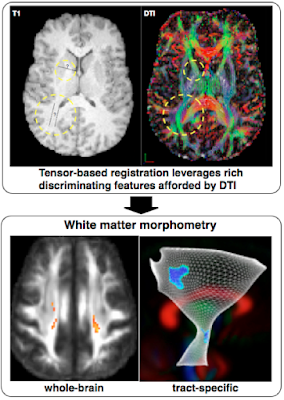Advantage of tensor specific normalization. Image by DTI-TK
In this tutorial there will be a number of steps that we have to run through in order to test statistical models, these steps are detailed below:
1. Make sure the tensor files are in the right format for tensor normalization.
2. Make population template by normalizing tensor images
3. Register population template to MNI space
4. Produce scalar images (FA, MD, AD, RD) and check quality
5. Run whole brain voxel-wise statistics

## 1. Standardize Tensor Images for Normalization

To get the tensor images ready for normalization we will have to make sure that tensors:
- have the right diffusivity unit,
- remove extreme outliers,
- and are symmetric and positive-definite.
These steps are described in detail on the website of DTI-TK; which is the website of the software package that we will use for the tensor normalization.

Example code:
```i=052212_s09_dti_eddy_fm_strip_dt.nii
prefix=052212_s09_dti_eddy_fm_strip
TVtool -in \$i -scale 1000000000 -out \${prefix}_sdt.nii.gz
echo ~~Checking for and removing Outliers~~
TVtool -in \${prefix}_sdt.nii.gz -norm
SVtool -in \${prefix}_sdt_norm.nii.gz -stats
BinaryThresholdImageFilter \${prefix}_sdt_norm.nii.gz \${prefix}_non_outliers.nii.gz 0 100 1 0
TVtool -in \${prefix}_sdt.nii.gz -mask \${prefix}_non_outliers.nii.gz -out \${prefix}_tmp.nii.gz
mv -f \${prefix}_tmp.nii.gz \${prefix}_sdt.nii.gz
TVtool -in \${prefix}_sdt.nii.gz -norm
echo ~Stats for\${prefix} - max should be below 100~
SVtool -in \${prefix}_sdt_norm.nii.gz -stats
echo ~~~Enforcing positive semi-definiteness~~~
TVtool -in \${prefix}_sdt.nii.gz -spd -out \${prefix}_tmp.nii.gz
mv -f \${prefix}_tmp.nii.gz \${prefix}_sdt.nii.gz
echo ~~~Standardizing Voxel Space~~~
TVAdjustVoxelspace -in \${prefix}_sdt.nii.gz -origin 0 0 0 -out \${prefix}_sdt.nii.gz
echo ~~~Resample to isotropic voxels~~~
TVResample -in \${prefix}_sdt.nii.gz -align center -size 256 256 128 -vsize 1 1 1
rm -f \${prefix}_tmp.nii.gz
rm -f \${prefix}_non_outliers.nii.gz
rm -f \${prefix}_sdt_nonSPD.nii.gz
rm -f \${prefix}_sdt_norm.nii.gz
```
Q1: Go through the DTI-TK preprocessing tutorial, indicate which lines of the above code correspond to which steps in the outline. What is the goal of each step? When you use this code make sure you use the right diffusivity units, the code above is just an example, find out how to convert your own here.

This code uses a number of new tools. One of which will be of interest to take a closer look at.

http://dti-tk.sourceforge.net/pmwiki/pmwiki.php?n=Documentation.TVtool

Q2: Using TVtool investigate how and then write the code to produce the axial diffusivity (AD) map from the standardized tensor file (download 052212_s09_dti_eddy_fm_strip_sdt.nii.gz here). What other maps can that tool help produce?

Q3: What are the 4 main diffusion measurements that are often used in DTI? Take a look at the explanation on this page, and describe which measure you would pick if you were selectively interested in white matter?

- Articles demonstrating improved registration when using DTI-TK here and here

## 2. Normalize Tensors Images

In the next step we will take the standardized tensor images for each individual subject and run a multi-step normalization. The steps of the normalization are detailed on the DTI-TK website, but essentially consists of four steps:
1. Make initial mean
2. Run a rigid registration
3. Run an affine registration
4. Run a diffeomorphic registration.
Each normalization step iteratively moves each individual image closer to the population mean. As described by DTI-TK:

"Rigid alignment aims to find a rigid transformation, a linear transformation that does not alter objects size and shape, that best aligns two objects in question. (...) Affine alignment improves upon rigid alignment by finding a more general linear transformation that allows objects' global size and shape to be altered. (...) After you've aligned your DTI volumes affinely, you can proceed with the deformable alignment, which is designed to improve alignment quality by removing size or shape differences between local structures." - DTI-TK
The below figure shows how the steps are applied and combined.

 Iterative normalization process. Image by DTI-TK
This process starts by averaging all the individual tensor images to create a starting point template, a population template that has yet to be normalized, the example FA from these tensor images may look something like this: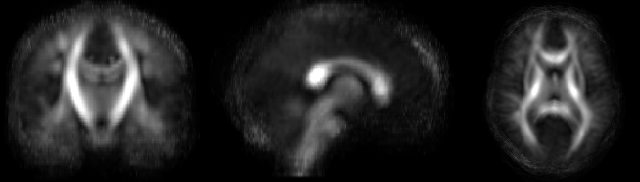Example of un-normalized average FA of population of images
Then as a next step each individual gets registered to this new population template iteratively. This process consists of rigid, affine and diffeomorphic registrations. A rigid-body transformation in three dimensions (x, y and z) is defined by six parameters: three translations and three rotations (source).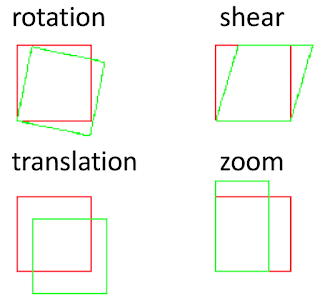Affine includes 12 parameter transformation: 3 rotations, 3 translations, 3 sheers, 3 zooms
These transformations are a subset of the 12 transformations allowed in an affine registration of a three dimensional volume, which besides the rotation and translation also includes three sheers, and three zooms. Although affine registrations still have the constraint that parallel lines remain parallel after registration, thus it is contained to a linear registration. Or as wiki puts it:
"In geometry, an affine transformation, affine map or an affinity (from the Latin, affinis, "connected with") is a function between affine spaces which preserves points, straight lines and planes. Also, sets of parallel lines remain parallel after an affine transformation. An affine transformation does not necessarily preserve angles between lines or distances between points, though it does preserve ratios of distances between points lying on a straight line." - Wikipedia
Finally the diffeomorphic or deformable registration removes those constraints too and allows for a non-linear higher dimension registration, as long as it is invertible.Examples of diffeomorphic transformations
Example script:
```echo ~~~Create an initial mean~~~;
for spd in `ls *sdt.nii.gz`;
do
subj_list_file=subj_list;
echo \$spd >> subj_list_file.txt;
done
TVMean -in \$subj_list_file.txt -out mean_initial.nii.gz;
TVResample -in mean_initial.nii.gz -vsize 1 1 1 -size 256 256 128;
echo ~~~Rigid alignment with initial mean~~~;
sh dti_rigid_population mean_initial.nii.gz \${subj_list_file}.txt EDS 3;
echo ~~~Affine alignment with output from rigid alignment~~~;
sh dti_affine_population mean_rigid3.nii.gz \${subj_list_file}.txt EDS 3;
echo ~~~Diffeomorphic alignment with output from affine alignment~~~;
TVtool -tr -in mean_affine3.nii.gz;
BinaryThresholdImageFilter mean_affine3_tr.nii.gz mask.nii.gz 0 .01 100 1 0;
sh dti_diffeomorphic_population mean_affine3.nii.gz \${subj_list_file}_aff.txt mask.nii.gz 0.002```

Q4: Which step do you think will take the most time to run? Note the step where you resample your image to have isotropic voxel size, which line is this? Read this post on the importance, explain why we need isotropic voxels for fiber tracking. Resampling also alters the image dimensions, when you change those, you can read here that DTI-TK needs your image dimensions to be powers of 2. What are most likely often used powers of 2 in neuroimaging volume dimensions?

- Courses on fMRI spatial normalization by Wellcome Trust centre here and here
- Courses on fMRI spatial normalization by SPM here
- Advanced normalization tools (ANTS) for normalization of scalar images
- Background documentation on normalization by ANTS
- Further documentation from DTI-TK on tensor normalization

## 3. Register population template to MNI-atlas

Below you can see the result of the population mean post-normalization. At this stage you are at a cross-roads of options how to analyze the results. The following steps are but one way, as you get more comfortable with analyses, software and scripting you can try to explore other options.Example of normalized average FA of population of images
After normalization you can register your population template to MNI space. For this step you can for example use one of the provided atlases in FSL, like the ICBM152, which is an average of 152 T1-weighted MRI scans, linearly and non-linearly (6 iterations) transformed to form a symmetric model (source). The template is shown below.MNI ICBM152 non-linear 6th generation. Image by FSL.
Registration software is notoriously bad at aligning images across modalities (like registering FA to T1). In this case, after many trial and errors, I have found that using asvDSM from DTI-TK has been best able to register FA files to T1 space, as you can see in the image below.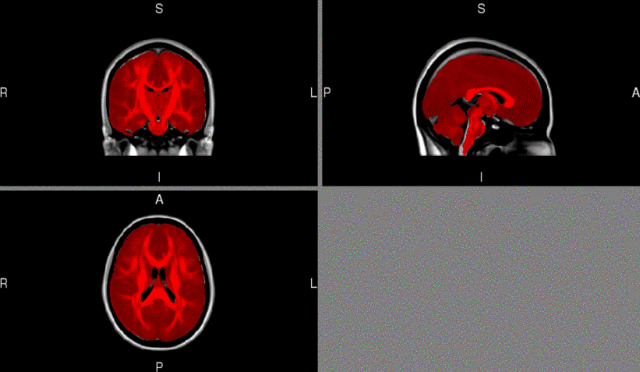Template FA (red) after registration to MNI space (gray-scale)
asvDSM by DTI-TK

Example script:
```echo ~~~Register population template to MNI~~~
TVtool -in \${population_tensor_mean} -tr -out my_tr.nii.gz
BinaryThresholdImageFilter my_tr.nii.gz my_mask.nii.gz 0.7 100 1 0
TVtool -in \${population_tensor_mean}_strip.nii.gz -fa -out \${population_tensor_mean}_strip_fa.nii.gz
asvDSM -template \${MNI_T1} -subject \${population_tensor_mean}_strip_fa.nii.gz -outTrans \${dtiToT1Trans} -sep 0.5 0.5 0.5 -ftol 0.0001
echo ~~~Run subject specific loop to warp to T1~~~
for i in `cat subjects.txt`;
do
echo ~Compose full warp and apply~
dfRightComposeAffine -aff \${subj}_affineTrans -df \${subj}_diffeoTrans -out \${subj}_combined.df.nii.gz
echo ~Combine full warp with affine to T1 and apply~
dfLeftComposeAffine -df \${subj}_combined.df.nii.gz -aff \${dtiToT1Trans} -out \${subj}_toT1_combined.df.nii.gz
deformationSymTensor3DVolume -in \${subj}_orig -trans \${subj}_toT1_combined.df.nii.gz -target \${T1} -out \${subj}_tensorFile
done
```
Q5: Open fslview, click "File" -> "Open standard". In this directory and in the directory atlases you can browse all the different atlases that are automatically loaded with FSL. You can also find the atlas images in your installed FSL directory. Find the MNI template that you would like to use for registration. What is the name of that file? Give 1 reason why you would want to register your data to this template.

- More on the available atlases in FSL
- More on the atlases by MNI
- White matter atlas by Hermoye et al.
- International consortium of brain mapping (ICBM) DTI-81 atlas
- Cortical association tracts by Mori et al. (2002)
- Fiber tract based atlas of human white matter by Wakana et al. (2004)
- A diffusion tensor tractography atlas by Catani & De Schotten (2008)
- More online atlases by JHU

## 4. Make scalars and check quality

As a next step we will make diffusion maps (FA, MD, AD, RD) of each individual in template space. These diffusion maps are also known as scalar images; they have magnitude but not direction. When you have those images you can go through them and get an impression of the quality of the normalization and your data. One way of quickly viewing all your images is by concatenating them into one 4 dimensional image using fslmerge.

fslmerge by FSL
http://fsl.fmrib.ox.ac.uk/fsl/fslwiki/Fslutils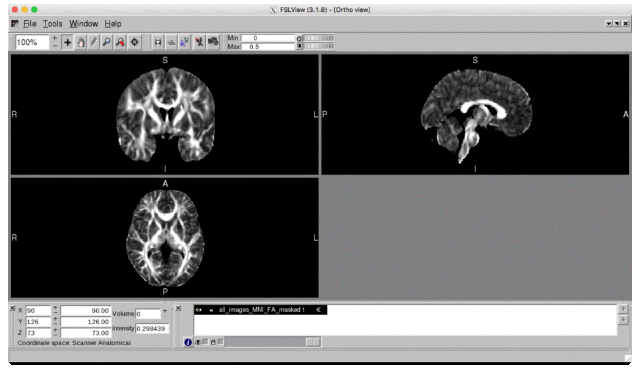Movie of normalized FA images
Example script:
```echo ~~~Produce subject specific smoothed scalars~~~
for subj in `cat subjects.txt`;
do
echo ~Produce diffusion maps~
TVtool -in \${subj}_tensorFile.nii.gz -fa -out \${subj}_fa.nii.gz
TVtool -in \${subj}_tensorFile.nii.gz -tr -out \${subj}_tr.nii.gz
TVtool -in \${subj}_tensorFile.nii.gz -rd -out \${subj}_rd.nii.gz
echo ~Smooth diffusion maps~
SVGaussianSmoothing -in \${subj}_fa.nii.gz -fwhm  4 4 4 -out \${subj}_fa4mm.nii.gz
SVGaussianSmoothing -in \${subj}_tr.nii.gz -fwhm  4 4 4 -out \${subj}_tr4mm.nii.gz
SVGaussianSmoothing -in \${subj}_rd.nii.gz -fwhm  4 4 4 -out \${subj}_rd4mm.nii.gz
done
echo ~~~Merge FA images for quality control~~~
fslmerge -t all_images_MNI_FA *_fa.nii.gz
```
Q6: Some of the code in this tutorial uses a "for loop" for the first time. Take a look at a tutorial on loops here. Try to figure out how "for loops" work, then describe what you would put in the subjects.txt file from line 2. HINT: Remember how variables work in bash. Another option is to run the below code, instead of line 2 and 3. Can you describe what this would do?
```for i in `ls *_diffeo_fa.nii.gz`;
do
subj=`echo \$i | awk 'BEGIN{FS="_diffeo_fa.nii.gz"}{print \$1}'````
Q7: Note that DTI-TK outputs the trace (TR) instead of the MD value. Look up how trace relates to MD here, and write the fslmaths code to convert TR to MD. If you are unsure how to do this, type fslmaths in your command line to see all the help options.

Q8: Next describe what lines 10-13 accomplish, if you are unsure read this documentation on spatial smoothing. Finally in line 16 we merge all the individual FA images, describe why you might want to do that.

- How to convert FWHM to sigma

## 5. Run whole brain voxel-wise statistics

Now that we have scalar images in normalized space for each individual we can run voxel-wise analyses. There is a plethora of tools that you can use to run your preferred statistical model. A method that is often used in diffusion imaging is tract-based spatial statistics (TBSS) by FSL. Although this method has become the somewhat accidental standard, it has recently come under some scrutiny. Bach et al. (2014) describe some important methodological considerations for using TBSS.  And Swartz et al. (2014) describe how TBSS's skeleton projection step actually reduces algorithm accuracy. This is due to developments in registration tools (e.g. tensor normalization with DTI-TK, and improved algorithms of advanced normalization tools [ANTS]), which has made the methods used in TBSS obsolete. Furthermore, there is the possibility that you miss subtle effects that are in one of the smaller tracts, that may get removed when the data is skeletonized.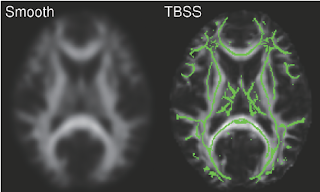Example of tract-based spatial statistics. Image by FSL
Due to these issues, I have a preference for running whole brain voxel-wise analyses. In this case I decided to use FSL's randomise, since it is free and does not depend on software that has to run in MatLab (like SPM and SurfStat). Randomise runs a non-parametric test, which means that it makes no assumptions about the underlying normality of the data, which is a more robust test than ordinary GLM's. If you read the randomise documentation you will also see that you can include covariates like age and sex. Which are important variables to include in anatomical studies.

randomise by FSL
http://fsl.fmrib.ox.ac.uk/fsl/fslwiki/Randomise/UserGuide

Example code:
```randomise -i all_merge_fa.nii.gz -o randomise_out -d test.mat -t test.con -m my_mask.nii.gz -T
```

Q9: Look at the user guide for randomise, and try to get an impression of what the .mat file and .con file contain. Produce an example of both for the imaginary case that you have a patient group (n=3) and healthy control group (n=3), and you want to test where FA is significantly lower in the patient group, and where FA is significantly lower in the control group. What does the option -T stand for in randomise?

Q10: Open fslview and open a standard 1mm MNI T1 image. Download example t-stat map (test_tstat1.nii.gz here), and add it to the MNI image. What you see is not yet very informative, click on the information dialog button (i in blue circle) and change the Lookup table options. Make the first Red-Yellow, and turn the second one on, and make it Blue-Lightblue. Close the dialog screen. Go to this p-value calculator, and input in the t-ratio boxes that you want a probability that is 0.05 and you have 5 degrees of freedom (number of subjects - 1). What is your 2-tailed significant t-statistic? Input that number in the fslview screen as a minimum brightness value, and make 5 your maximum brightness value. Although this is obviously just test data, you now see what a noisy (when you only test 6 subjects you will most likely only find noise) t-stat map can look like. It is now up to you to start using your own data and improve on this! Remember to correct for multiple comparison when running your data to avoid inflated false discovery rates. You are running a t-tests on each individual voxel, which combine to multiple thousands per brain, and you will have to correct for this. Read more about familywise error rates here. What does the -T option do to help with this issue? HINT: Read this .pdf on the background of threshold-free cluster enhancement (TFCE).

- YouTube channel on analysis of neuroimaging data by Jeanette Mumford- Tutorial on including voxel-wise covariates using SurfStat
- Stanford university information on voxel-wise tensor statistics and whole brain diffusion stats
- Wikipedia on false discovery rate (FDR)
- Lecture on Multiple comparison issues from Columbia University
- Genovese et al. (2002) on FDR for neuroimaging
- Brainvoyager software on multiple comparison correction and FDR
- More info on thresholding by the late Keith Worthsley, and the seminal publication on significance testing in MRI by Worthsley et al. (1996)

When you are done with your analyses and want to share your statistical maps publicly, you can use NeuroVault. Sharing your statistical maps will help improve meta analyses, collaborations and science in general.Upload your unthresholded statistical map here, and share your URL in your publication

---------------------
Reference this post as: Do Tromp, DTI Tutorial 2 - Normalization and Statistics, The Winnower 3:e145653.31599 (2016). DOI: 10.15200/winn.145653.31599

### The diffusion tensor, and its relation to FA, MD, AD and RD

In DTI 101 we described how diffusion tensor imaging estimates brain microstructure by modeling diffusion of water in the brain. We briefly discuss that this is done by using ellipsoid or ball shaped tensors. This leads to the question; what is a tensor?

"Tensors are simply mathematical objects that can be used to describe physical properties, just like scalars and vectors." - University of Cambridge
Or, more specifically as described by Kahn Academy:

In the scanner the diffusion tensor is measured by imaging the diffusion in individual gradient directions, like in the image below. If you are interested a more in depth mathematical explanation of this principle is provided in our post here.Example work out for 6 gradient directions, from diffusion image to tensor
Once the water diffusion is measured there are a number of ways you can quantify the shape of the tensors in each voxel. In DTI there are 4 measures that are most commonly used; fractional anisotropy, mean diffusivity, axial diffusivity and radial diffusivity. These measure directly relate to the value of the three main eigenvalues of the tensor, indicated in the figure below with lambda 1lambda 2 and lambda 3. What is an eigenvalue of a tensor? It is the value of the displacement/diffusion for each specific vector. Or, as defined by wikipedia:

"If a two-dimensional space is visualized as a rubber sheet, a linear map with two eigenvectors and associated eigenvalues λ1 and λ2 may be envisioned as stretching/compressing the sheet simultaneously along the two directions of the eigenvectors with the factors given by the eigenvalues." - Wikipedia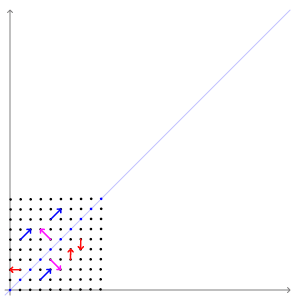Example of the influence of the transformation (or in our case diffusion) on the eigenvalues
There are roughly two archetypical examples of diffusion tensors. On the left you see the example where the tensor has roughly equal eigenvalues for each main vector, thus showing an isotropic diffusion profile. On the right an example of diffusion primarily in one direction, thus demonstrating an anisotropic diffusion profile.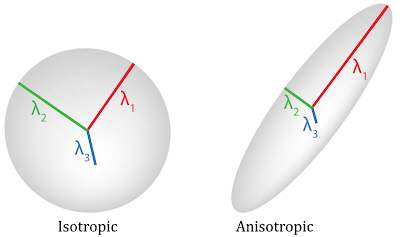Examples of tensor shapes
Characterization of each eigenvalue in the tensor and combinations of them help constitute the main diffusion measures. Specifically, in axial diffusivity (AD) we only quantify the value of lambda 1. While in radial diffusivity (RD) we take the average of lambda 2 and lambda 3. Mean diffusivity (MD) provides an average of all three, lambda 1, lambda 2, and lambda 3, this measure is sometimes also referred to as trace (TR), which is the sum of lambda 1lambda 2 and lambda 3. And finally fractional anisotropy (FA) provides the relative difference between the largest eigenvalue as compared to the others; it quantifies the fraction of diffusion that is anisotropic. The exact mathematical relations are shown below: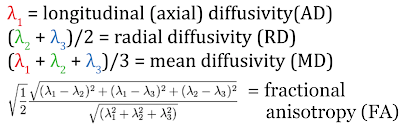Relation of diffusivity measures to the eigenvalues of the tensor
When you plot the value of each a diffusivity measure in every voxel you can get scalar maps of the quantification of the local tensors. In the image below you see representative examples of each measure. When you look at the MD map it becomes apparent that the mean diffusivity measure is specifically sensitive to cerebral spinal fluid (CSF), which has high values of average diffusion. In voxels with much CSF mean diffusivity is high, and values are thus bright. While AD is only sensitive to diffusion in the longest eigenvalue. Here you see that highly organized structures like white matter pathways are brights. In addition large open cavities like ventricles have general high levels of diffusion which translates to high lambda 1 values. Then RD represents the two shortest eigenvalues and shows dark values in highly organized and dense structures like white matter pathways, intermediate values in gray matter, and high values in regions with CSF. Finally, FA plots the relative length of lambda 1, compared to lambda 2 and lambda 3. This leads to selective brightness in white matter, but not gray matter or CSF.Examples of each diffusivity measure, FA, MD, AD and RD